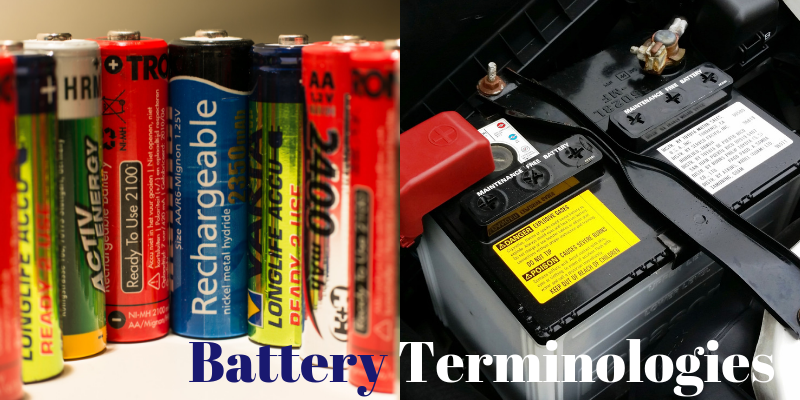Energy Storage

# An introduction to battery terminologies

Batteries are finding newer applications and usage these days. With the advent of electric vehicles and large-scale energy storage plans, the need to bring out newer battery technologies and improvisation has become paramount importance. While there are a lot of improvisation going on, there is a need to understand the various terminologies that define the battery capacity. The conversion of chemical energy and vice versa happens inside a battery. The various parameters involved in battery are described below.

Basics

Battery Assembly

A battery may consist of several cells, packs or modules. The cell is the smallest form of battery. A collection of cells grouped together is called modules. A group of modules is called pack. The grouping is done by series or parallel or a combination of series and parallel connection.

Classification

Batteries can be classified as High Power or High Energy. It can be either of these but not both. There are also high durable battery which has longer life time at the cost of power and energy.

C – Rate

The C rate denotes the charge rate and discharge rate. Batteries usually come in C 1 rating, C 5 rating, C 10 rating and C 20 rating. C 1 rating means, the battery can be charged at 100A in one hour and discharged in one hours. For example, a 100Ah Battery can be charged in one hour at 100A and can be discharge 100A in one hour. In case of a C 5 rated battery, the 100Ah battery can be charged in 5 hours at 20A per hour, while the same can be discharged in 5 hours at 5A per hours. In case of C 20 rating, a 100 Ah battery will be charged at 5A per hour in 20 hours. The same can be discharged at 5A per hour in 20 hours. Usually Lithium batteries are C 1 rated. VRLA and Lead Acid batteries are C 10 and C 20 rated. C10 is used in solar applications, while C20 is used in UPS and domestic inverter applications.

Cells

Cells are classified as Primary and Secondary Cells. Primary batteries are not rechargeable. They can be used only once. Secondary batteries are rechargeable batteries. They can be used over and over.

Battery Terminologies

State of Charge percentage (SOC %)

The percentage of present charge that the battery has when compared to its full capacity is SOC. The current level is used to calculate the SOC.

Depth of Discharge percentage (DoD %)

Depth of discharge is the percentage of discharge that has happened when compared to full capacity. Discharge beyond 80% is usually called deep discharge.

Terminal Voltage (V)

The terminal voltage is the voltage measured at the battery terminals when connected to load. It varies based on state of charge percentage and discharge rate.

Open-Circuit Voltage (V)

The terminal voltage is the voltage measured at the battery terminals when the battery is not connected to load. It varies based on state of charge percentage.

Internal Resistance

Internal resistance is the resistance within the battery. It is, generally different for charging and discharging. It also depends on the state of charge. Battery efficiency decreases with the increase of internal resistance. This decreases the thermal stability as most of the energy will be lost as heat.

Battery Technical Specifications

Nominal Voltage (V)

The voltage stated in the data sheet also called reference voltage or normal voltage.

Cut off voltage (V)

The voltage below which the battery should be discharged is the cut of voltage.

Capacity

It is also called the nominal capacity. It is calculated by multiplying the discharge current in ampere with the discharge time in hours. It is mentioned in Ah. It is the amp hours available when the battery is discharged at the predetermined C rate.

Energy or Nominal Energy

It is calculated my multiplying the ampere hour with the battery nominal voltage at the predetermined C rate. It is mentioned in Watt Hour.

Life Cycle

One Charge and discharge is called one once cycle. The number of charges and discharges that can be performed at a specific C rate is called the Life Cycle. Higher DoD, lower is the life cycle of the battery.

Specific Energy (Wh / Kg)

The nominal battery energy per unit mass is called the specific energy.

Specific Power (W/ Kg)

Specific power is the maximum power available per unit mass.

Energy Density (Wh/L)

It is also called volumetric energy density. It is the nominal battery energy per unit volume.

Power Density (W/L)

Power density is the maximum available power per unit volume.

Maximum Continuous Discharge Current

It is the maximum current at which the battery can be discharged continously.

Float Voltage

After being charged to 100%, batteries undergo self-discharge to keep up the state of charge. The voltage at that level is called float voltage.

Call us